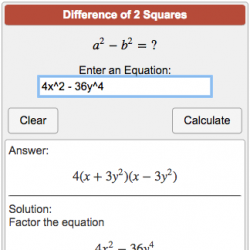## Factoring Trinomials | Calculator with various steps, examples & solutions

The Factoring Trinomials Calculator is a free online calculator that shows the factors of a particular trinomial. However, a trinomial is a polynomial with only three terms in mathematics. Variables,…